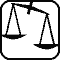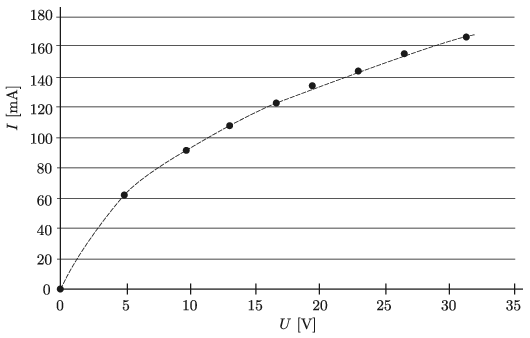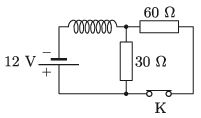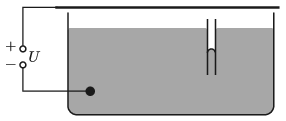Mathematical and Physical Journal
for High Schools
Issued by the MATFUND Foundation
 Already signed up? New to KöMaL?

# KöMaL Problems in Physics, January 2022

Show/hide problems of signs:## Problems with sign 'M'

Deadline expired on February 18, 2022.

M. 410. If a playing card is placed between a small, strong magnet and a horizontal paper clip, then we can lift the paper clip by the card. Measure how many cards you need to stack so that you can no longer lift the paper clip. What is the thickness of these stacked sheets? Connect two magnets of the same size and investigate how many cards are needed so that we can no longer lift the paper clip.

(6 pont)

statistics## Problems with sign 'G'

Deadline expired on February 18, 2022.

G. 765. Each of the 11 shots in the series of photos was taken from the same location, with the camera always facing the Sun. The chronological order of the photos is from left to right. What was the time when the image of the Sun was the closest to the horizon? To which point of the compass did the camera face when the Sun was at its lowest position in the sky? Where and in which season was the series of photos taken?(4 pont)

solution (in Hungarian), statistics

G. 766. The most famous formula in physics is the relation of $\displaystyle E=mc^2$ expressing the equivalence of mass and energy where $\displaystyle E$ is the energy, $\displaystyle m$ is the mass, and $\displaystyle c$ is the speed of light in vacuum. Using this, estimate how much heavier is our mobile phone, when its battery is fully charged, than when its battery is fully discharged.

(3 pont)

solution (in Hungarian), statistics

G. 767. The Morning Star (actually the planet Venus) is visible for a while only in the evenings, and then it appears for a while only at dawns. What is the period of this change?

(4 pont)

solution (in Hungarian), statistics

G. 768. The figure shows the current-voltage characteristic of one of the tungsten filament bulbs of a string of a Christmas tree lights, which contains a hundred bulbs connected in series.$\displaystyle a)$ Using the graph determine the total dissipated electrical energy by all the bulbs of the string if it is connected to a voltage supply of 230V.

$\displaystyle b)$ What is the total dissipated electrical energy by the string if it contains only ten bulbs and it is connected to 230 V?

Note: In the second case the bulbs will quite soon burn out.

(4 pont)

solution (in Hungarian), statistics## Problems with sign 'P'

Deadline expired on February 18, 2022.

P. 5373. The 7.3 km M4 metro line connects Kelenföld railway station and Keleti railway station, and between them it has 8 other stops. Trains leave each station (or stop) with a constant acceleration of 1.0 m/s$\displaystyle {}^2$ and and stop with the same deceleration when braking. The maximum speed between stations is 80 km/h. At the stations the average time to change passengers is 0.5 minutes.

$\displaystyle a)$ How long does it take for the train to reach its cruising speed when it leaves the station? How far does it travel during this time?

$\displaystyle b)$ During one journey, how long does the train travel at the speed of 80 km/h?

$\displaystyle c)$ What is the journey time of the M4 metro between the two terminals, i.e. how much time elapses between the departure of a train from Kelenföld and its arrival at Keleti railway station?

(4 pont)

solution (in Hungarian), statistics

P. 5374. The picture shows a serial firing, spring-loaded toy rifle, which can fire six thin, cylindrical sponge bullets. Before each shot, the black slide must be pulled to the rightmost position by about 10 cm. We used a digital scale and found that the maximum force required to cock the rifle is the same as the weight of a 6.6 kg object.$\displaystyle a)$ How could the force have been determined if no auxiliary means other than the balance was used?

$\displaystyle b)$ Estimate the maximum velocity at which a sponge bullet of mass 3 g will fly out if 10% of the energy stored in the spring is used to accelerate the bullet.

(4 pont)

solution (in Hungarian), statistics

P. 5375. One end of a thin, uniform-density stick lying in a frictionless horizontal plane is suddenly struck so that the the velocity of the end point is perpendicular to the stick and has a magnitude of $\displaystyle v$.

$\displaystyle a)$ Which part of the stick will have zero initial speed?

$\displaystyle b)$ What is the speed and direction of the motion of the other end of the stick, at the moment when it starts to move?

(5 pont)

solution (in Hungarian), statistics

P. 5376. A horizontal tank of length $\displaystyle 2L$ is divided into into two parts, having equal volume, by a thermally insulating piston. Both parts contain a sample of ideal gas of $\displaystyle n$ moles of diatomic molecules at a temperature of $\displaystyle T_0$. To each side of the piston a horizontal spring of spring constant $\displaystyle D$ is attached. Both springs are designed for compression and tension and their other ends are fixed to the vertical walls of the container. The springs are initially unstretched. When the gas in the right part of the container is slowly heated, the piston moves a distance of $\displaystyle L/2$ towards the left. During the process the gas in the left part is connected to a high heat capacity heat reservoir of temperature $\displaystyle T_0$.

$\displaystyle a)$ What is the pressure of the gas in the right part when the piston has moved a distance of $\displaystyle x$ from the original position?

$\displaystyle b)$ Determine the heat absorbed by the gas in the right part during the whole process.

(5 pont)

solution (in Hungarian), statistics

P. 5377. Three point-like objects, each having an electric charge of $\displaystyle q$, are fixed at the vertices of an equilateral triangle. Another point-like object of charge $\displaystyle Q$ is placed to the centroid of the triangle. What should the charge $\displaystyle Q$ of this fourth object be in order that even if the fixation of the objects is ceased all of the charged objects remain at rest?

(4 pont)

solution (in Hungarian), statistics

P. 5378. Switch K in the circuit shown in the figure has been closed for a long time. Once the switch is opened. What is the magnitude of the induced electromotive force in the coil immediately after the switch is opened?(5 pont)

solution (in Hungarian), statistics

P. 5379. With the help of ideal polarizing filters, we would like to turn the polarization plane of linearly polarized light by $\displaystyle 45^\circ$ in order to reduce the intensity loss by at most 10%. At least how many polarizing filters are needed and how should they be optimally arranged?

(5 pont)

solution (in Hungarian), statistics

P. 5380. In a special isotope laboratory, extreme activity $\displaystyle {}^{137}$Cs and $\displaystyle {}^{60}$Co sources are used to validate dosimeters. When the two high-purity radioactive sources were checked, it was found that in a minute approximately the same number of decays occurred in the case of the 68 mg caesium source, and in the case of the cobalt source of unknown mass.

$\displaystyle a)$ What is the mass of the cobalt source?

$\displaystyle b)$ After how long and which source will have twice the activity of the other? (Half-life of $\displaystyle {}^{137}$Cs: 30.17 years, half-life of $\displaystyle {}^{60}$Co: 5.27 years.)

(4 pont)

solution (in Hungarian), statistics

P. 5381. An (insulating) glass container is filled with mercury. A vertical capillary tube of diameter $\displaystyle d=0.5$ mm is immersed in the mercury as shown in the figure. Above the surface of the mercury at a height of $\displaystyle h=6$ mm, a large, horizontal metal sheet is placed. How much does the mercury level in the capillary tube change if a DC voltage source of $\displaystyle U=20$ kV is connected across the mercury and the metal sheet?(6 pont)

solution (in Hungarian), statistics